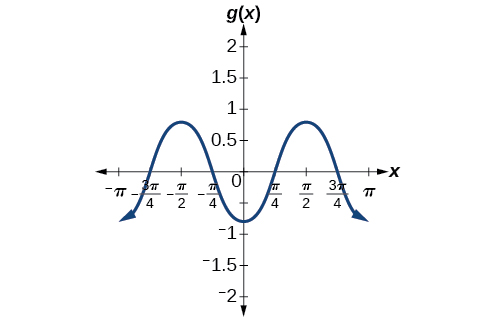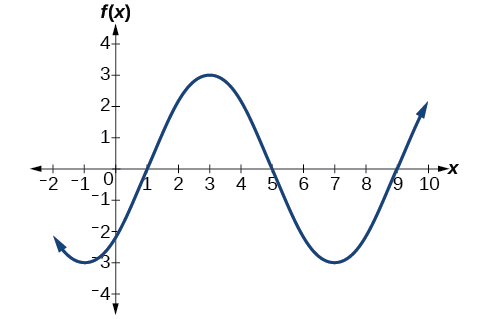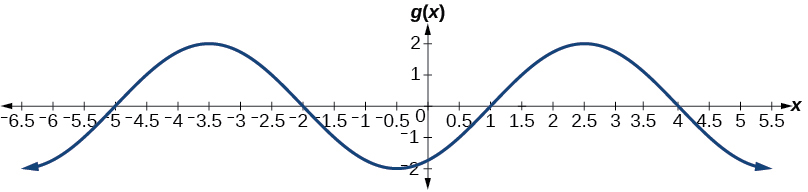# 6.1 Graphs of the sine and cosine functions  (Page 5/13)

 Page 5 / 13

Write a formula for the function graphed in [link] .

two possibilities: $\text{\hspace{0.17em}}y=4\mathrm{sin}\left(\frac{\pi }{5}x-\frac{\pi }{5}\right)+4\text{\hspace{0.17em}}$ or $\text{\hspace{0.17em}}y=-4\mathrm{sin}\left(\frac{\pi }{5}x+\frac{4\pi }{5}\right)+4$

## Graphing variations of y = sin x And y = cos x

Throughout this section, we have learned about types of variations of sine and cosine functions and used that information to write equations from graphs. Now we can use the same information to create graphs from equations.

Instead of focusing on the general form equations

we will let $\text{\hspace{0.17em}}C=0\text{\hspace{0.17em}}$ and $\text{\hspace{0.17em}}D=0\text{\hspace{0.17em}}$ and work with a simplified form of the equations in the following examples.

Given the function $\text{\hspace{0.17em}}y=A\mathrm{sin}\left(Bx\right),\text{\hspace{0.17em}}$ sketch its graph.

1. Identify the amplitude, $\text{\hspace{0.17em}}|A|.$
2. Identify the period, $\text{\hspace{0.17em}}P=\frac{2\pi }{|B|}.$
3. Start at the origin, with the function increasing to the right if $\text{\hspace{0.17em}}A\text{\hspace{0.17em}}$ is positive or decreasing if $\text{\hspace{0.17em}}A\text{\hspace{0.17em}}$ is negative.
4. At $\text{\hspace{0.17em}}x=\frac{\pi }{2|B|}\text{\hspace{0.17em}}$ there is a local maximum for $\text{\hspace{0.17em}}A>0\text{\hspace{0.17em}}$ or a minimum for $\text{\hspace{0.17em}}A<0,\text{\hspace{0.17em}}$ with $\text{\hspace{0.17em}}y=A.$
5. The curve returns to the x -axis at $\text{\hspace{0.17em}}x=\frac{\pi }{|B|}.$
6. There is a local minimum for $\text{\hspace{0.17em}}A>0\text{\hspace{0.17em}}$ (maximum for $\text{\hspace{0.17em}}A<0$ ) at $\text{\hspace{0.17em}}x=\frac{3\pi }{2|B|}\text{\hspace{0.17em}}$ with $\text{\hspace{0.17em}}y=–A.$
7. The curve returns again to the x -axis at $\text{\hspace{0.17em}}x=\frac{\pi }{2|B|}.$

## Graphing a function and identifying the amplitude and period

Sketch a graph of $\text{\hspace{0.17em}}f\left(x\right)=-2\mathrm{sin}\left(\frac{\pi x}{2}\right).$

Let’s begin by comparing the equation to the form $\text{\hspace{0.17em}}y=A\mathrm{sin}\left(Bx\right).$

• Step 1. We can see from the equation that $\text{\hspace{0.17em}}A=-2,$ so the amplitude is 2.
$|A|=2$
• Step 2. The equation shows that $\text{\hspace{0.17em}}B=\frac{\pi }{2},\text{\hspace{0.17em}}$ so the period is
• Step 3. Because $\text{\hspace{0.17em}}A\text{\hspace{0.17em}}$ is negative, the graph descends as we move to the right of the origin.
• Step 4–7. The x -intercepts are at the beginning of one period, $\text{\hspace{0.17em}}x=0,\text{\hspace{0.17em}}$ the horizontal midpoints are at $\text{\hspace{0.17em}}x=2\text{\hspace{0.17em}}$ and at the end of one period at $\text{\hspace{0.17em}}x=4.$

The quarter points include the minimum at $\text{\hspace{0.17em}}x=1\text{\hspace{0.17em}}$ and the maximum at $\text{\hspace{0.17em}}x=3.\text{\hspace{0.17em}}$ A local minimum will occur 2 units below the midline, at $\text{\hspace{0.17em}}x=1,\text{\hspace{0.17em}}$ and a local maximum will occur at 2 units above the midline, at $\text{\hspace{0.17em}}x=3.\text{\hspace{0.17em}}$ [link] shows the graph of the function.

Sketch a graph of $\text{\hspace{0.17em}}g\left(x\right)=-0.8\mathrm{cos}\left(2x\right).\text{\hspace{0.17em}}$ Determine the midline, amplitude, period, and phase shift.midline: $\text{\hspace{0.17em}}y=0;\text{\hspace{0.17em}}$ amplitude: $\text{\hspace{0.17em}}|A|=0.8;\text{\hspace{0.17em}}$ period: $\text{\hspace{0.17em}}P=\frac{2\pi }{|B|}=\pi ;\text{\hspace{0.17em}}$ phase shift: $\text{\hspace{0.17em}}\frac{C}{B}=0\text{\hspace{0.17em}}$ or none

Given a sinusoidal function with a phase shift and a vertical shift, sketch its graph.

1. Express the function in the general form
2. Identify the amplitude, $\text{\hspace{0.17em}}|A|.$
3. Identify the period, $\text{\hspace{0.17em}}P=\frac{2\pi }{|B|}.$
4. Identify the phase shift, $\text{\hspace{0.17em}}\frac{C}{B}.$
5. Draw the graph of $\text{\hspace{0.17em}}f\left(x\right)=A\mathrm{sin}\left(Bx\right)\text{\hspace{0.17em}}$ shifted to the right or left by $\text{\hspace{0.17em}}\frac{C}{B}\text{\hspace{0.17em}}$ and up or down by $\text{\hspace{0.17em}}D.$

## Graphing a transformed sinusoid

Sketch a graph of $\text{\hspace{0.17em}}f\left(x\right)=3\mathrm{sin}\left(\frac{\pi }{4}x-\frac{\pi }{4}\right).$

• Step 1. The function is already written in general form: $\text{\hspace{0.17em}}f\left(x\right)=3\mathrm{sin}\left(\frac{\pi }{4}x-\frac{\pi }{4}\right).$ This graph will have the shape of a sine function    , starting at the midline and increasing to the right.
• Step 2. $\text{\hspace{0.17em}}|A|=|3|=3.\text{\hspace{0.17em}}$ The amplitude is 3.
• Step 3. Since $\text{\hspace{0.17em}}|B|=|\frac{\pi }{4}|=\frac{\pi }{4},\text{\hspace{0.17em}}$ we determine the period as follows.
$P=\frac{2\pi }{|B|}=\frac{2\pi }{\frac{\pi }{4}}=2\pi \cdot \frac{4}{\pi }=8$

The period is 8.

• Step 4. Since $\text{\hspace{0.17em}}C=\frac{\pi }{4},\text{\hspace{0.17em}}$ the phase shift is
$\frac{C}{B}=\frac{\frac{\pi }{4}}{\frac{\pi }{4}}=1.$

The phase shift is 1 unit.

• Step 5. [link] shows the graph of the function.A horizontally compressed, vertically stretched, and horizontally shifted sinusoid

Draw a graph of $\text{\hspace{0.17em}}g\left(x\right)=-2\mathrm{cos}\left(\frac{\pi }{3}x+\frac{\pi }{6}\right).\text{\hspace{0.17em}}$ Determine the midline, amplitude, period, and phase shift.midline: $\text{\hspace{0.17em}}y=0;\text{\hspace{0.17em}}$ amplitude: $\text{\hspace{0.17em}}|A|=2;\text{\hspace{0.17em}}$ period: $\text{\hspace{0.17em}}P=\frac{2\pi }{|B|}=6;\text{\hspace{0.17em}}$ phase shift: $\text{\hspace{0.17em}}\frac{C}{B}=-\frac{1}{2}$

difference between calculus and pre calculus?
give me an example of a problem so that I can practice answering
x³+y³+z³=42
Robert
dont forget the cube in each variable ;)
Robert
of she solves that, well ... then she has a lot of computational force under her command ....
Walter
what is a function?
I want to learn about the law of exponent
explain this
what is functions?
A mathematical relation such that every input has only one out.
Spiro
yes..it is a relationo of orders pairs of sets one or more input that leads to a exactly one output.
Mubita
Is a rule that assigns to each element X in a set A exactly one element, called F(x), in a set B.
RichieRich
If the plane intersects the cone (either above or below) horizontally, what figure will be created?
can you not take the square root of a negative number
No because a negative times a negative is a positive. No matter what you do you can never multiply the same number by itself and end with a negative
lurverkitten
Actually you can. you get what's called an Imaginary number denoted by i which is represented on the complex plane. The reply above would be correct if we were still confined to the "real" number line.
Liam
Suppose P= {-3,1,3} Q={-3,-2-1} and R= {-2,2,3}.what is the intersection
can I get some pretty basic questions
In what way does set notation relate to function notation
Ama
is precalculus needed to take caculus
It depends on what you already know. Just test yourself with some precalculus questions. If you find them easy, you're good to go.
Spiro
the solution doesn't seem right for this problem
what is the domain of f(x)=x-4/x^2-2x-15 then
x is different from -5&3
Seid
All real x except 5 and - 3
Spiro
***youtu.be/ESxOXfh2Poc
Loree
how to prroved cos⁴x-sin⁴x= cos²x-sin²x are equal
Don't think that you can.
Elliott
By using some imaginary no.
Tanmay
how do you provided cos⁴x-sin⁴x = cos²x-sin²x are equal
What are the question marks for?
ElliottByByBy Vanessa SoledadBy Katy KeilersBy Michael SagBy OpenStaxBy Stephen VoronBy Prateek AshtikarBy Mahee BooBy Christine ZeelieBy OpenStaxBy Brooke Delaney